Williamson matrices

A Hadamard matrix of orderis an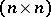-matrixwith as entriesandsuch that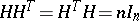, where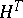is the transposed matrix ofand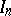is the unit matrix of order. Note that the problem of constructing Hadamard matrices of all orders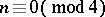is as yet unsolved (1998; the first open case is). For a number of methods for constructing Hadamard matrices of concrete orders, see [a1], [a9], [a7]. One of these methods, described below, is due to J. Williamson [a10]. Let,,, andbe pairwise commuting symmetric circulant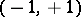-matrices of ordersuch that(such matrices are called Williamson matrices). Then the Williamson array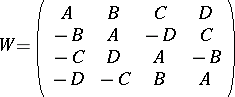is a Hadamard matrix of order. The recent achievements about the construction of Hadamard matrices are connected with the construction of orthogonal designs [a4] (cf. also Design with mutually orthogonal resolutions), Baumert–Hall arrays [a2], Goethals–Seidel arrays [a5] and Plotkin arrays [a6], and with the construction of Williamson-type matrices, i.e., of four or eight-matrices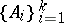,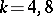, of orderthat satisfy the following conditions:

i)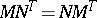,;

ii). Williamson-four matrices have been constructed for all orders, with the exception of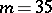, which was eliminated by D.Z. Djokovic [a3], by means of an exhaustive computer search. It is worth mentioning that Williamson-type-four matrices of orderare not yet known (1998). Williamson-four and Williamson-type-four matrices are known for many values of. For details, see [a9], Table A1; pp. 543–547. The most recent results can be found in [a11].

There are known Williamson-type-eight matrices of the orders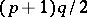, where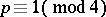,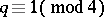are prime numbers [a8].

A set of-matrices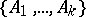is called a Williamson family, of type, if the following conditions are fulfilled:

a) There exists a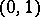-matrix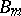of ordersuch that for arbitrary,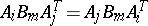;

b). If, then the typeis denoted by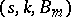.

If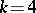,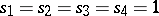, and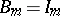, then each Williamson family of typecoincides with a family of Williamson-type matrices.

If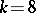,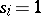for, and, then each Williamson family of type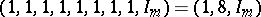coincides with a family of Williamson-type-eight matrices.

If,, and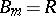,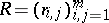,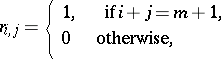and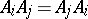, then each Williamson family of type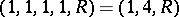coincides with a family of generalized Williamson-type matrices.

An orthogonal design of orderand type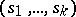(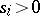) on commuting variablesis an-matrixwith entries from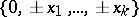such thatLetbe a Williamson family of type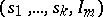and suppose there exists an orthogonal design of typeand orderthat consists of elements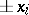,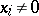. Then there exists a Hadamard matrix of order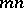. In other words, the existence of orthogonal designs and Williamson families implies the existence of Hadamard matrices. For more details and further constructions see [a4], [a9].